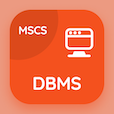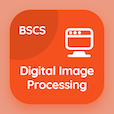Computer Science Online Courses

Digital Logic Design Quizzes

Digital Logic Design Practice Test 16

The e-Book Introduction to Lab Experiments Quiz Questions, introduction to lab experiments MCQ with answers PDF chapter 8-16 to learn online courses, digital logic design tests. Practice DLD Lab Equipment and Experiments trivia questions, introduction to lab experiments Multiple Choice Questions (MCQ Quiz) for online college degrees. The e-Book "Introduction to Lab Experiments Quiz" App Download: introduction to lab experiments, introduction to combinational logics, rectangular shape symbols, shift registers, introduction to msi and pld components test prep for top computer science schools.

The Quiz "In the 14 pin gate, PIN 14 is marked" PDF, Introduction to Lab Experiments App Download (Free) with vdd, vcc, ground, and ac choices for online college courses. Solve dld lab equipment and experiments questions and answers, Amazon eBook to download free sample for computer science associate degree.

## Logic Design: Introduction to Lab Experiments Quiz

MCQ: In the 14 pin gate, PIN 14 is marked

A) Vcc
B) Vdd
C) ground
D) AC

MCQ: Circuits that employ memory elements in addition to gates is called

A) combinational circuit
B) sequential circuit
C) combinational sequence
D) series

MCQ: Converting code X to code Y is represented by the symbol of

A) X+Y
B) X-Y
C) X*Y
D) X/Y

MCQ: When J and complement of K are 1, flip-flop QA after the shift is equal to

A) 1
B) 0
C) reset
D) defined

MCQ: PLD stands for

A) Portable Large Device
B) Portable Logic Device
C) Programmable Large Device
D) Programmable Logic Device

### Mock Tests: Digital Logic Design Course Prep

Download Digital Logic Design Quiz App, DataBase Management System (MCS) MCQs App and Digital Image Processing MCQ App for Android & iOS devices. These Apps include complete analytics of real time attempts with interactive assessments. Download Play Store & App Store Apps & Enjoy 100% functionality with subscriptions!